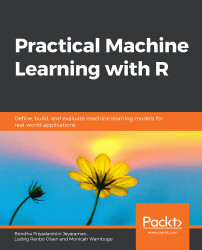•#### Practical Machine Learning with R#### Overview of this book

With huge amounts of data being generated every moment, businesses need applications that apply complex mathematical calculations to data repeatedly and at speed. With machine learning techniques and R, you can easily develop these kinds of applications in an efficient way. Practical Machine Learning with R begins by helping you grasp the basics of machine learning methods, while also highlighting how and why they work. You will understand how to get these algorithms to work in practice, rather than focusing on mathematical derivations. As you progress from one chapter to another, you will gain hands-on experience of building a machine learning solution in R. Next, using R packages such as rpart, random forest, and multiple imputation by chained equations (MICE), you will learn to implement algorithms including neural net classifier, decision trees, and linear and non-linear regression. As you progress through the book, you’ll delve into various machine learning techniques for both supervised and unsupervised learning approaches. In addition to this, you’ll gain insights into partitioning the datasets and mechanisms to evaluate the results from each model and be able to compare them. By the end of this book, you will have gained expertise in solving your business problems, starting by forming a good problem statement, selecting the most appropriate model to solve your problem, and then ensuring that you do not overtrain it.Free Chapter
An Introduction to Machine LearningData Cleaning and Pre-processingFeature EngineeringIntroduction to neuralnet and Evaluation MethodsLinear and Logistic Regression ModelsUnsupervised LearningAppendix## Summary

In this chapter, we fitted and interpreted multiple linear and logistic regression models. We learned how to calculate the RMSE and MAE metrics, and checked their different responses to outliers. We generated model formulas and cross-validated them with the cvms package. To check whether our model is better than random guesses and making the same prediction every time, we created baseline evaluations for both linear regression and binary classification tasks. When multiple metrics (such as the F1 score and balanced accuracy) disagree on the ranking of models, we learned to find the nondominated models, also known as the Pareto front. Finally, we trained two random forest models and compared them to the best performing linear and logistic regression models.

In the next chapter, you will learn about unsupervised learning.